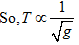×#### Thank you for registering.

One of our academic counsellors will contact you within 1 working day.

Click to Chat

1800-1023-196

+91-120-4616500

CART 0

• 0

MY CART (5)

Use Coupon: CART20 and get 20% off on all online Study Material

ITEM
DETAILS
MRP
DISCOUNT
FINAL PRICE
Total Price: Rs.

There are no items in this cart.
Continue Shopping
```
Considering the elastic and the inertial aspects involved, explain the fact that whereas when an object of mass m oscillates vertically on a spring the period depends on m but is independent of g, the reverse is true for a simple pendulum.

```
5 years ago

```							The force constant k of a spring is numerically equal to the force required to produce a unit extension on it. Whenever a spring is extended or compressed, a restoring force comes into play and acts in a direction opposite to the direction of increasing displacement.The time period (T) of vibrations varies inversely as the square root of the force constant (k) of the spring.The time period (T) of vibrations varies directly as the square root of the mass (m) of body attached to the string.Thus the time period of an object of mass m oscillates vertically on a spring is independent of acceleration due to gravity g and is given by,So, T = 2π √m/kSimple pendulum consists of a heavy metallic bob, suspended from a rigid support by means of a long, weightless, flexible and inextensible string. Dependence of time period, of a simple pendulum, on various factors is explained by the laws of simple pendulum.Law of isochromism:- It states that for a simple amplitude (≤4° ), the time period of a simple pendulum is independent of its amplitude.Law of length:- It states that time period of a simple pendulum varies directly as the square root of its length.So,Law of acceleration due to gravity:- It states that the time period of a simple pendulum varies inversely as the square root of acceleration due to gravity at that place.Thus the time period of a simple pendulum depends upon acceleration due to gravity g and is given by,T = 2π √l/gTherefore from the above observation we conclude that, when an object of mass m oscillates vertically on a spring the period depends on m but is independent of g, but the reverse is true for a simple pendulum.
```
5 years ago
```							Thus the time period of a simple pendulum depends upon acceleration due to gravity g and is given by,T = 2p vl/gTherefore from the above observation we conclude that, when an object of mass m oscillates vertically on a spring the period depends on m but is independent of g, but the reverse is true for a simple pendulum.The force constant k of a spring is numerically equal to the force required to produce a unit extension on it. Whenever a spring is extended or compressed, a restoring force comes into play and acts in a direction opposite to the direction of increasing displacement.The time period (T) of vibrations varies inversely as the square root of the force constant (k) of the spring.The time period (T) of vibrations varies directly as the square root of the mass (m) of body attached to the string.Thus the time period of an object of mass m oscillates vertically on a spring is independent of acceleration due to gravity g and is given by,So, T = 2p vm/kSimple pendulum consists of a heavy metallic bob, suspended from a rigid support by means of a long, weightless, flexible and inextensible string. Dependence of time period, of a simple pendulum, on various factors is explained by the laws of simple pendulum.Law of isochromism:- It states that for a simple amplitude (=4° ), the time period of a simple pendulum is independent of its amplitude.Law of length:- It states that time period of a simple pendulum varies directly as the square root of its length.So,Law of acceleration due to gravity:- It states that the time period of a simple pendulum varies inversely as the square root of acceleration due to gravity at that place.
```
5 years ago
Think You Can Provide A Better Answer ?

## Other Related Questions on Wave Motion

View all Questions »### Course Features

• 101 Video Lectures
• Revision Notes
• Previous Year Papers
• Mind Map
• Study Planner
• NCERT Solutions
• Discussion Forum
• Test paper with Video Solution### Course Features

• 110 Video Lectures
• Revision Notes
• Test paper with Video Solution
• Mind Map
• Study Planner
• NCERT Solutions
• Discussion Forum
• Previous Year Exam Questions